# Warm up Solve the given system by substitution

• Slides: 15Warm up Solve the given system by substitution: 1) 2 x – y = 7 3 x + 3 y = - 3 Solve the given system by elimination: 2) -3 x + 4 y = -4 3 x – 6 y = 6Summary of Methods 1) Substitution: Requires that one of the variables be isolated on one side of the equation. It is especially convenient when one of the variables has a coefficient of 1 or -1. 2) Elimination: Can be applied to any system, but it is especially convenient when a variable appears in different equations with coefficients that are opposites. 3) Graphing: Can provide a useful method for estimating a solution.What is the Best Method for the following? 1. y = 4 x – 3 5 x – 2 y = 6 2. 4 x – 5 y = 13 2 x + 5 y = 5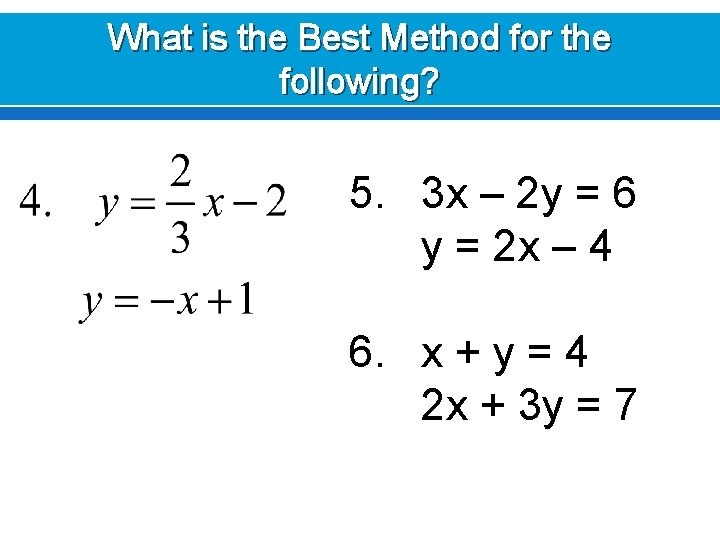What is the Best Method for the following? 5. 3 x – 2 y = 6 y = 2 x – 4 6. x + y = 4 2 x + 3 y = 7Solving Word Problems Using Systems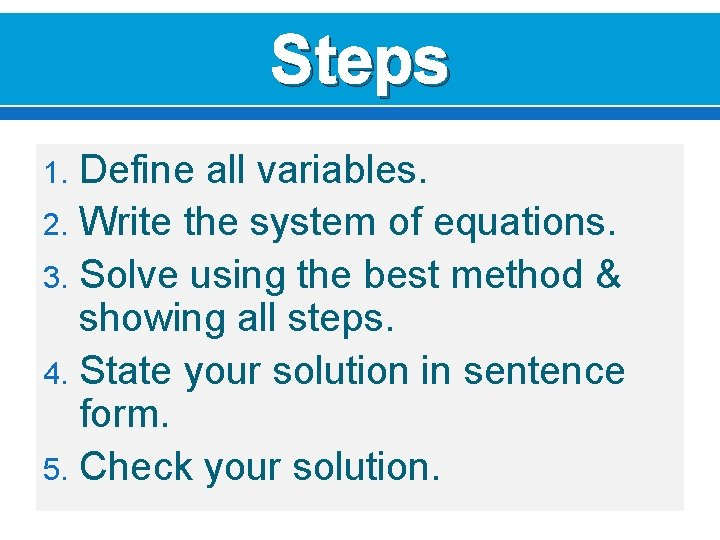Steps Define all variables. 2. Write the system of equations. 3. Solve using the best method & showing all steps. 4. State your solution in sentence form. 5. Check your solution. 1.1. You are selling tickets for a high school basketball game. Student tickets cost \$3 and general admission tickets cost \$5. You sell 350 tickets and collect \$1450. How many of each type of ticket did you sell?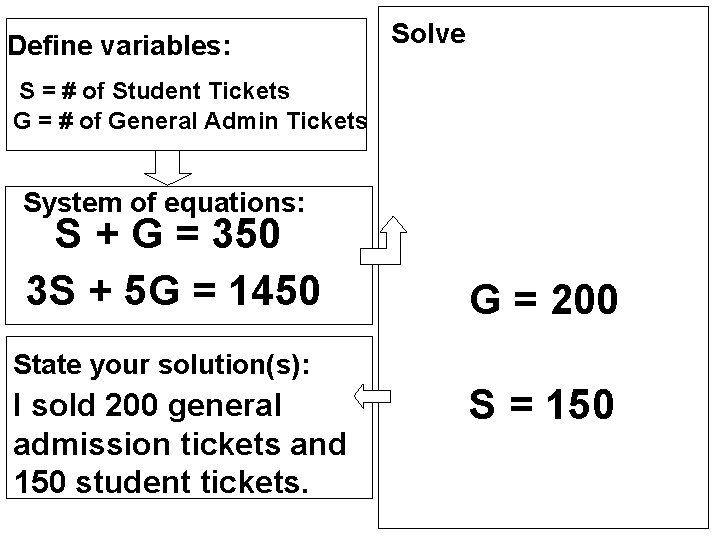Define variables: Solve S = # of Student Tickets G = # of General Admin Tickets System of equations: S + G = 350 3 S + 5 G = 1450 G = 200 State your solution(s): I sold 200 general admission tickets and 150 student tickets. S = 1502. Simon invests \$1200 into two savings accounts. One account earns 4% annual interest and the other earns 5. 9% annual interest. At the end of 1 year, Simon earned \$64. 15 in interest. How much did he invest at each rate?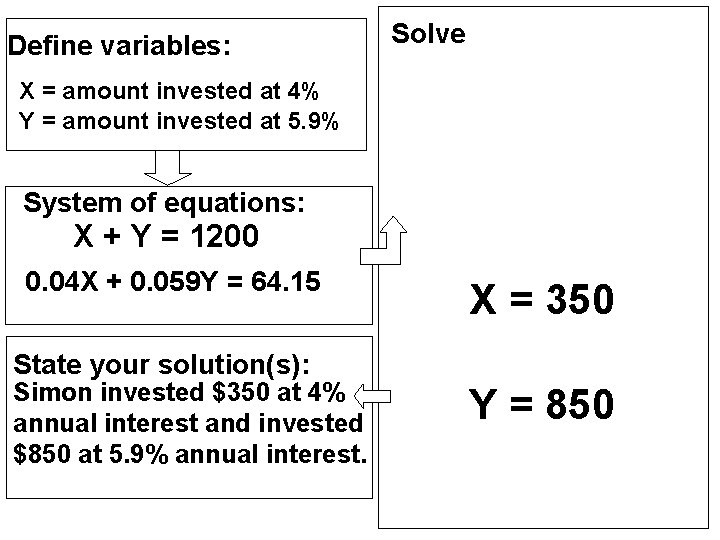Define variables: Solve X = amount invested at 4% Y = amount invested at 5. 9% System of equations: X + Y = 1200 0. 04 X + 0. 059 Y = 64. 15 X = 350 State your solution(s): Simon invested \$350 at 4% annual interest and invested \$850 at 5. 9% annual interest. Y = 8503. At an Italian bistro, the costs of 2 plates of spaghetti and 1 salad is \$27. 50. The cost for 4 plates of spaghetti and 3 salads is \$59. 50. Find the cost of a plate of spaghetti and a salad.Define variables: Solve P = cost plate of spaghetti S = cost salad System of equations: 2 P + S = 27. 50 4 P + 3 S = 59. 50 P = 11. 50 State your solution(s): A plate of spaghetti costs \$11. 50 and a salad costs \$4. 50. S = 4. 50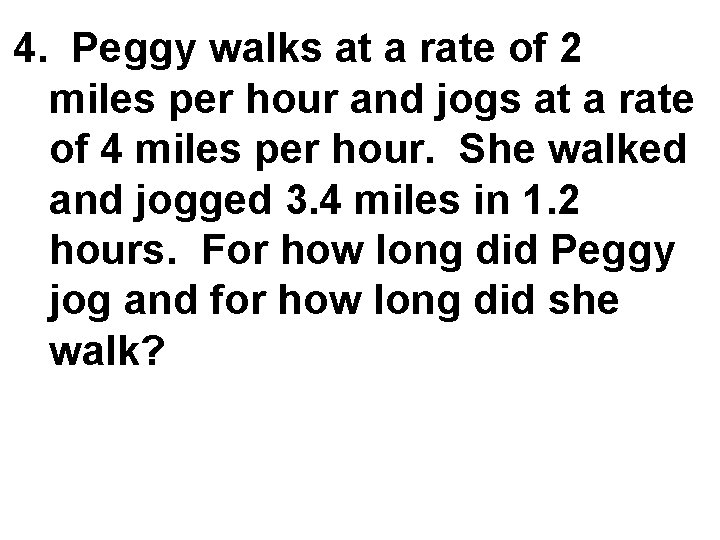4. Peggy walks at a rate of 2 miles per hour and jogs at a rate of 4 miles per hour. She walked and jogged 3. 4 miles in 1. 2 hours. For how long did Peggy jog and for how long did she walk?Define variables: Solve W = hours walked J = hours jogged System of equations: W + J = 1. 2 2 W + 4 J = 3. 4 W =. 7 State your solution(s): Peggy walked for 0. 7 hours and jogged for 0. 5 hours. J =. 5Homework Exit Ticket Thursday, Nov. 2 nd Worksheet Systems of Equations Word Problems # 1 ( 1 – 6 ) Group A Systems of Equations Word Problems #2 (1 -6) Group B I-Ready Lessons (minimum of 2 lessons and a quiz with at least 45 minutes or more for the week)